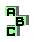STEINER INELLIPSE

 r43 point X(115), ninecircle d Steiner axis r152 isogonal points r153 homothetic triangles r204 duality, point X(190), incircle, ninecircle, complement r412 Euler line, cevian quotient, centroid r441 centroid, antipedal triangle r576 Steiner circumellipse r650 medial triangle, intouch triangle, side triangle, perspective r684 tripole, Gergonne point, circumconic, Soddy points r696 pedal triangle, cevian triangle, perspective, side triangle, circumcenter r886 tripolar r1036 r1037 centroid, circumcevian triangle r1113 isotomic points, third Brocard point r1165 isotomic complement, tripolar r1173 inconic, perspector, circumconic r1210 centroid, pedal triangle r1211 complement, Steiner circumellipse r1227 r1262 duality, anticomplement, symmedian point, orthic axis r1371 circumconic,perspector r1375 tripolar, perspector r1669 centroid, tripolar, anticevian triangle r1809 incircle, perspective